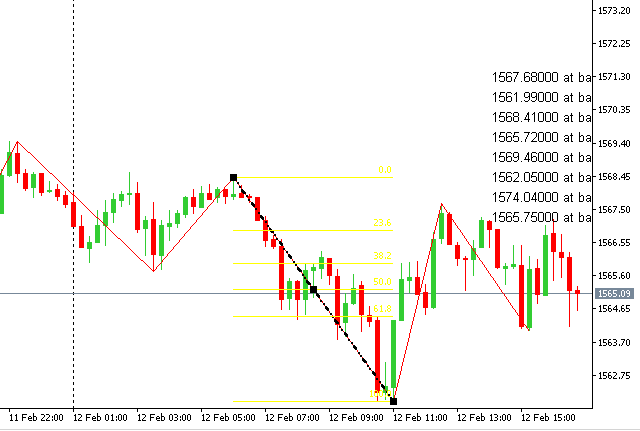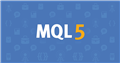# How can I change Fibonacci levels display colour?

I tried to change the Fibonacci display color but it only changes the color of the diagonal line across the levels as in the image below. How can I change the level colors? Please see my relevant code below:``` //+------------------------------------------------------------------+
//| Create Fibonacci Retracement by the given coordinates            |
//+------------------------------------------------------------------+
bool FiboLevelsCreate(const long            chart_ID=0,        // chart's ID
const string          name="FiboLevels", // object name
const int             sub_window=0,      // subwindow index
datetime              time1=0,           // first point time
double                price1=0,          // first point price
datetime              time2=0,           // second point time
double                price2=0,          // second point price
const color           clr=clrBlack,        // object color
const ENUM_LINE_STYLE style=STYLE_SOLID, // object line style
const int             width=1,           // object line width
const bool            back=false,        // in the background
const bool            selection=true,    // highlight to move
const bool            ray_left=false,    // object's continuation to the left
const bool            ray_right=false,   // object's continuation to the right
const bool            hidden=true,       // hidden in the object list
const long            z_order=0)         // priority for mouse click
{
//--- set anchor points' coordinates if they are not set
ChangeFiboLevelsEmptyPoints(time1,price1,time2,price2);
//--- reset the error value
ResetLastError();
//--- Create Fibonacci Retracement by the given coordinates
if(!ObjectCreate(chart_ID,name,OBJ_FIBO,sub_window,time1,price1,time2,price2))
{
Print(__FUNCTION__,
": failed to create \"Fibonacci Retracement\"! Error code = ",GetLastError());
return(false);
}
//--- set color
ObjectSetInteger(chart_ID,name,OBJPROP_COLOR,clr);
//--- set line style
ObjectSetInteger(chart_ID,name,OBJPROP_STYLE,style);
//--- set line width
ObjectSetInteger(chart_ID,name,OBJPROP_WIDTH,width);
//--- display in the foreground (false) or background (true)
ObjectSetInteger(chart_ID,name,OBJPROP_BACK,back);
//--- enable (true) or disable (false) the mode of highlighting the channel for moving
//--- when creating a graphical object using ObjectCreate function, the object cannot be
//--- highlighted and moved by default. Inside this method, selection parameter
//--- is true by default making it possible to highlight and move the object
ObjectSetInteger(chart_ID,name,OBJPROP_SELECTABLE,selection);
ObjectSetInteger(chart_ID,name,OBJPROP_SELECTED,selection);
//--- enable (true) or disable (false) the mode of continuation of the object's display to the left
ObjectSetInteger(chart_ID,name,OBJPROP_RAY_LEFT,ray_left);
//--- enable (true) or disable (false) the mode of continuation of the object's display to the right
ObjectSetInteger(chart_ID,name,OBJPROP_RAY_RIGHT,ray_right);
//--- hide (true) or display (false) graphical object name in the object list
ObjectSetInteger(chart_ID,name,OBJPROP_HIDDEN,hidden);
//--- set the priority for receiving the event of a mouse click in the chart
ObjectSetInteger(chart_ID,name,OBJPROP_ZORDER,z_order);
//--- successful execution
return(true);
}

//+------------------------------------------------------------------+
//| Check the values of Fibonacci Retracement anchor points and set  |
//| default values for empty ones                                    |
//+------------------------------------------------------------------+
void ChangeFiboLevelsEmptyPoints(datetime &time1,double &price1,
datetime &time2,double &price2)
{
//--- if the second point's time is not set, it will be on the current bar
if(!time2)
time2=TimeCurrent();
//--- if the second point's price is not set, it will have Bid value
if(!price2)
price2=SymbolInfoDouble(Symbol(),SYMBOL_BID);
//--- if the first point's time is not set, it is located 9 bars left from the second one
if(!time1)
{
//--- array for receiving the open time of the last 10 bars
datetime temp;
CopyTime(Symbol(),Period(),time2,10,temp);
//--- set the first point 9 bars left from the second one
time1=temp;
}
//--- if the first point's price is not set, move it 200 points below the second one
if(!price1)
price1=price2-200*SymbolInfoDouble(Symbol(),SYMBOL_POINT);
}```

You need to add the code to the help (OBJ_FIBO). Like this:

```//--- create an object
if(!FiboLevelsCreate(0,InpName,0,date[d1],price[p1],date[d2],price[p2],InpColor,
InpStyle,InpWidth,InpBack,InpSelection,InpRayLeft,InpRayRight,InpHidden,InpZOrder))
{
return;
}
//---
int levels                 = 3;                                   // number of level lines
double values           = {0.0,0.236,0.382};                   // values of level lines
color colors            = {clrAliceBlue,clrBlue,clrRed};       // color of level lines
ENUM_LINE_STYLE styles  = {STYLE_SOLID,STYLE_DASH,STYLE_DOT};  // style of level lines
int widths              = {1,2,3};                             // width of level lines
long chart_ID              = 0;                                   // chart's ID
string name                = "FiboLevels";                        // object name
FiboLevelsSet(levels,      // number of level lines
values,      // values of level lines
colors,      // color of level lines
styles,      // style of level lines
widths,      // width of level lines
chart_ID=0,  // chart's ID
name);
//--- redraw the chart and wait for 1 second
ChartRedraw();
Sleep(1000);```Documentation on MQL5: Constants, Enumerations and Structures / Objects Constants / Object Types / OBJ_FIBO
• www.mql5.com
//| Create Fibonacci Retracement by the given coordinates            |               time1=0,                            price1=0,                         time2=0,                            price2=0,                        width=1,            //